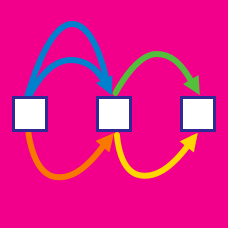Discrete Mathematics

# Rule of Sum and Rule of Product: Level 1 Challenges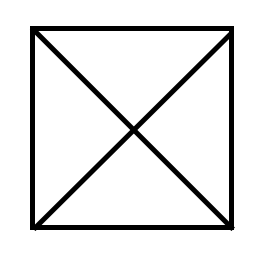How many triangles can you find in this figure?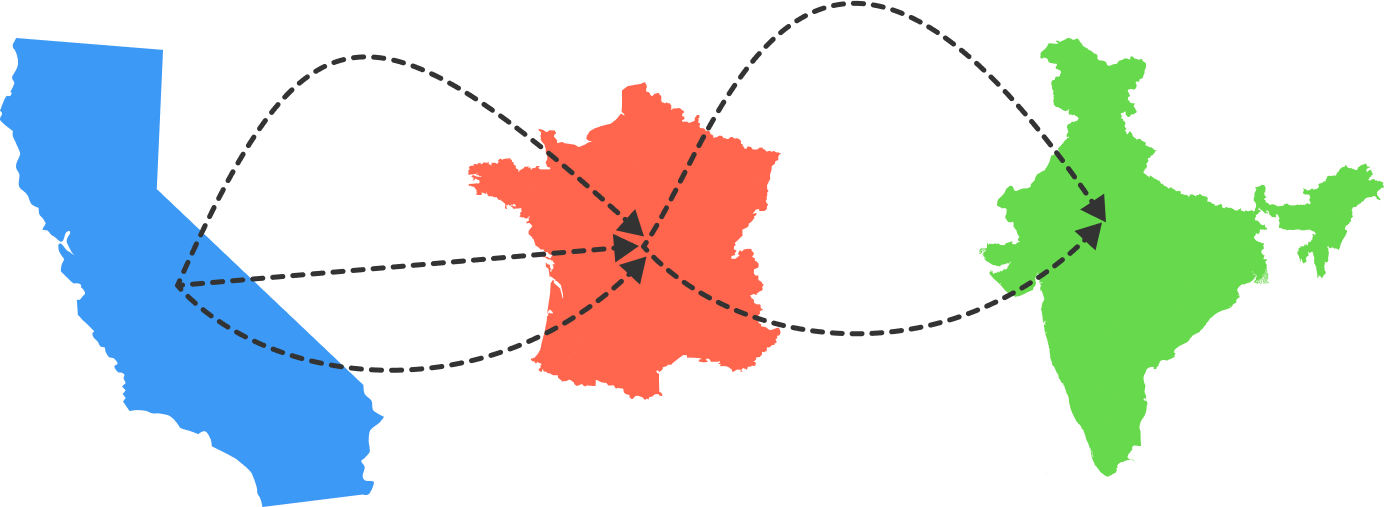There are 3 flights from California to France, and 2 flights from France to India. Sanjeet wants to fly from California to France and then to India.

How many choices does he have for his flight plan?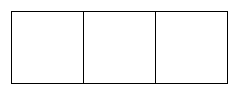We can color each square red, blue, or green. How many ways are there to color the squares such that any 2 adjacent squares are different colors?

For example, these are valid: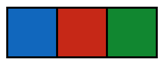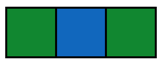but these are not: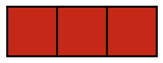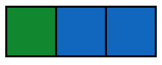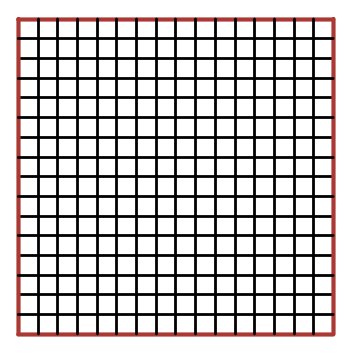If an $$n \times n$$ grid composed of unit squares has a red perimeter, and exactly 44 of the unit squares have exactly one red side, how long are the sides of the $$n \times n$$ square?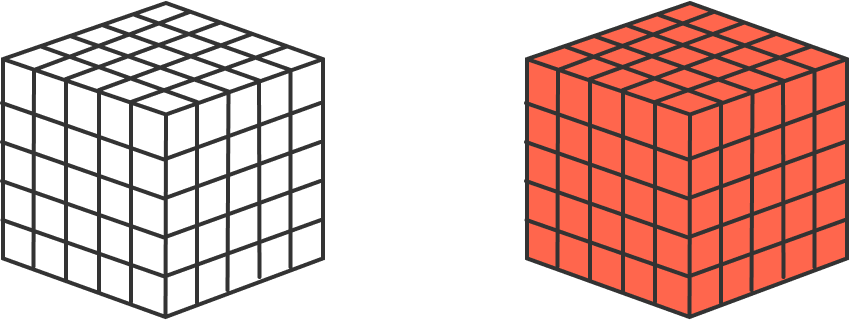If someone painted the outside of a $$5 \times 5 \times 5$$ cube made out of $$1 \times 1 \times 1$$ unit cubes, how many unit cubes would have paint on exactly 2 sides?

×

Problem Loading...

Note Loading...

Set Loading...